Disclaimer: This is an example of a student written essay.

Any opinions, findings, conclusions or recommendations expressed in this material are those of the authors and do not necessarily reflect the views of UKEssays.com.

# Mathematical Modelling Report

 ✅ Paper Type: Free Essay ✅ Subject: Mathematics ✅ Wordcount: 7845 words ✅ Published: 18th May 2020

Scientific problems are approached by building ‘models’. Not little cardboard models, but models made from mathematical tools. We can play with the symbolic models and adjust them until they start to behave in a way that resembles the way we expect them to, in order to be able to make projections. When we have done this, we get an understanding of the things we care about which is much deeper than we could ever get if we only use words and pictures. Mathematical models do not replace words and pictures, they sharpen them. So models deepen our understanding of ‘systems’, whether we are talking about a mechanism, a robot, a chemical plant, an economy, a virus, an ecology, a cancer or a brain. And it is necessary to understand something about how models are made.

In the following tasks, we are going to use such approach, in which we apply calculus to solve these tasks, and try to understand to behaviour of the functions asserted, or evolved from our analytical approach.

Given that the Rock ’n’ Roll coaster given over interval of 8 seconds, then by inspection:

$h\left(8\right)=–4{\left(8\right)}^{3}+46{\left(8\right)}^{2}–118×8+48=0$

Also, given that the Colossos coaster is modelled by:

$f\left(t\right)={t}^{3}–13{t}^{2}+44t–32$

Which is also modelled for 8 seconds, so:

$f\left(8\right)={8}^{3}–13{\left(8\right)}^{2}+44×8–32=0$

Hence, by the Factor Theorem, (t-8) must be a factor of both f(t) and h(t).

First, we divide h(t) by (t-8) as follows: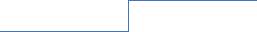$–4{t}^{2}+14t–6$

$t–8$

$–4{t}^{3}+46{t}^{2}–118t+48$$–4{t}^{3}+32{t}^{2}$

$14{t}^{2}–118t+48$$14{t}^{2}–112t$

$–6t+48$$–6t+48$

0

Therefore:

$h\left(t\right)=\left(t–8\right)\left(–4{t}^{2}+14t–6\right)$

$=–2\left(t–8\right)\left(2t–1\right)\left(t–3\right)$

Which shows that the Rock ‘n’ Roll coaster will reach the ground level at t=0.5s, t=3s, and t=8s.Similarly, dividing f(t) by (t-8) gives:        ${t}^{2}–5t+4$

$t–8$${t}^{3}–8{t}^{2}$

$–5{t}^{2}+44t–32$$–5{t}^{2}+40t$

$4t–32$$4t–32$

0

Which means that:

$f\left(t\right)=\left(t–8\right)\left({t}^{2}–5t+4\right)$

$f\left(t\right)=\left(t–1\right)\left(t–4\right)\left(t–8\right)$

Hence the Colossos reaches the ground level at t=1s, t=4s, and t=8s.

To find maximum and minimum heights we use the first and second derivatives as follows:

1)      The Rock ‘n’ Roll coaster:

${h}^{‘}\left(t\right)=–12{t}^{2}+92t–118$

${h}^{‘}\left(t\right)=0$

$–12{t}^{2}+92t–118=0$

$t=\frac{–92±\sqrt{2800}}{–24}=\frac{23}{6}\mp \frac{5}{6}\surd 7$

So, for:

${t}_{1}=\frac{23}{6}–\frac{5}{6}\sqrt{7}\approx 1.63s$

we have $h\left({t}_{1}\right)=–39.45$

And for:

${t}_{2}=\frac{23}{6}+\frac{5}{6}\sqrt{7}\approx 6.04s$

we have $h\left({t}_{2}\right)=132.04$

To determine the nature of these stationary points we apply the second derivative test as follows:

$h‘‘\left(t\right)=–24t+92$

so, at the point (1.63, -39.45) we have a minimum since:

${h}^{‘‘}\left(1.63\right)=–24×1.63+92=52.88>0$

Similarly, at the point (6.04, 132.04) the coaster reaches a maximum point since:

${h}^{‘‘}\left(6.04\right)=–24×6.04+92=–52.96<0$

2)      The Colossos coaster:

${f}^{‘}\left(t\right)=3{t}^{2}–26t+44$

${f}^{‘}\left(t\right)=0$

$3{t}^{2}–26t+44=0$

$t=\frac{26±\sqrt{148}}{6}=\frac{13}{3}±\frac{\sqrt{37}}{3}$

So, for:

${t}_{1}=\frac{13}{3}–\sqrt{37}/3\approx 2.31s$

we have $f\left({t}_{1}\right)=12.60$

And for:

${t}_{2}=\frac{13}{3}+\sqrt{37}/3\approx 6.04s$

we have $f\left({t}_{2}\right)=–20.75$

To determine the nature of these stationary points we apply the second derivative test as follows:

${f}^{‘‘}\left(t\right)=6t–26$

so, at the point (2.31, 12.60) we have a maximum since:

${f}^{‘‘}\left(2.31\right)=2.31×6–26=–12.14<0$

Similarly, at the point (6.36, -20.75) the coaster reaches a minimum point since:

${f}^{‘‘}\left(6.04\right)=6×6.36–26=12.16>0$

Table of values for both graphs are as follows:

 t h(t) 0 48 0.5 -28 1 0 2 -36 3 0 4 56 5 108 6 132 6.5 126 7 104 7.5 63 8 0
 t f(t) 0 -32 1 0 2 12 3 10 4 0 5 -12 6 -20 7 -18 8 0

And the graphs of both h(t) and f(t) are shown below:

h(t)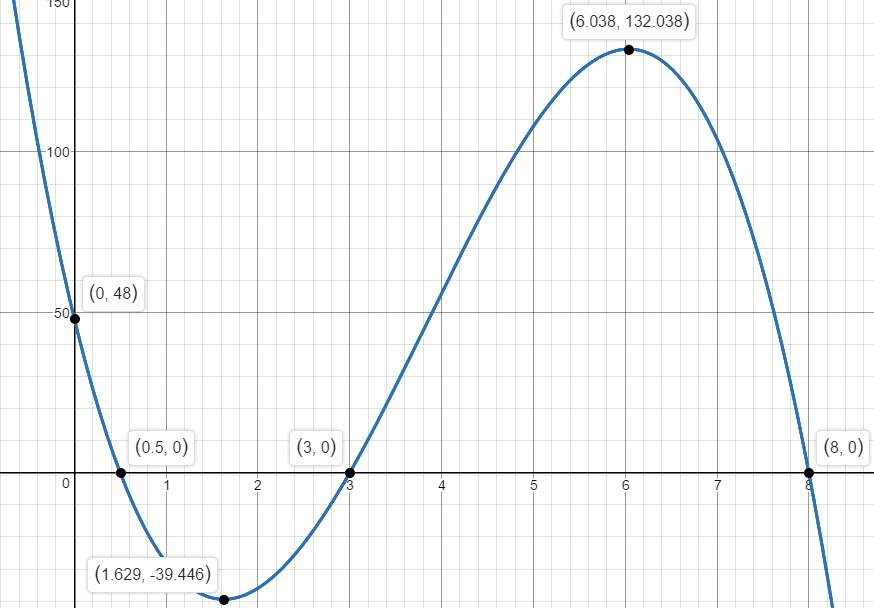f(t)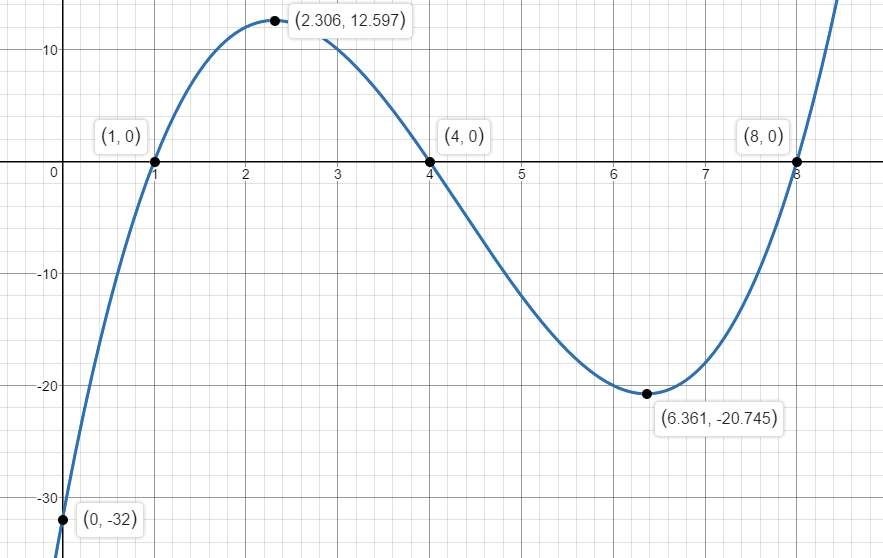The above graphs clearly show that the Rock ‘n’ Roll coaster is bigger than the Colossos coaster.

Finally, to determine which coaster has the larger underground area, we calculate the areas underneath the x-axis for both functions as follows:

• The Rock ‘n’ Roll coaster:

• The Colossos coaster:

${\int }_{0}^{1}\left({t}^{3}–13{t}^{2}+44t–32\right)\mathit{dt}+{\int }_{4}^{8}\left({t}^{3}–13{t}^{2}+44t–32\right)\mathit{dt}={\left[\frac{{t}^{4}}{4}–\frac{13}{3}{t}^{3}+22{t}^{2}–32t\right]}_{0,1}+{\left[\frac{{t}^{4}}{4}–\frac{13}{3}{t}^{3}+22{t}^{2}–32t\right]}_{4,8}\approx 67.42$

Therefore, we can deduce that the Colossos coaster has larger underground area than the Rock ‘n’ Roll coaster.

To find the maximum speed we use the first derivative in order to find the local points, as follows:

${v}^{‘}\left(t\right)=3–0.4t$

And setting the first derivative equal to zero gives:

$3–0.4t=0$

Or                                                                   $t=\frac{3}{0.4}=7.5s$

Which gives:

$v\left(7.5\right)=3\left(7.5\right)–0.2{\left(7.5\right)}^{2}=11.25m{s}^{–1}$

And to prove that this speed is a maximum speed we apply the second derivative test as follows:

So, the stationary point found is a maximum.

And a graph of the given velocity model is shown below: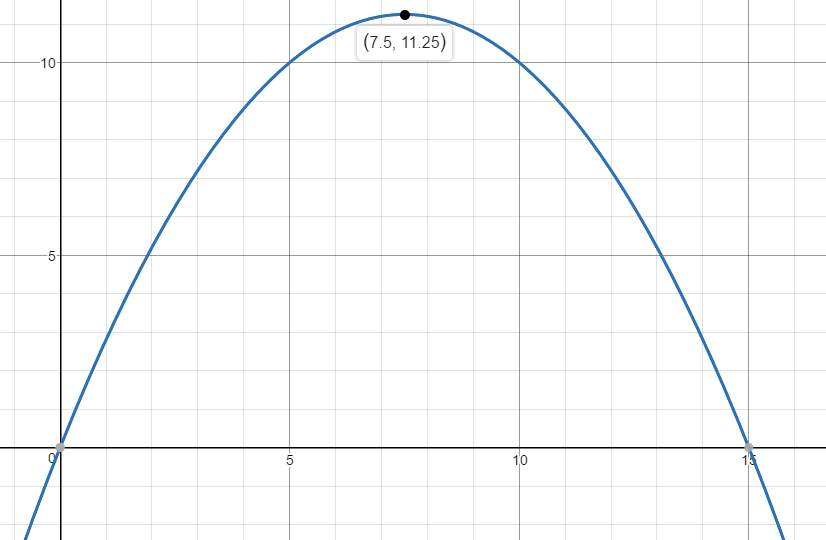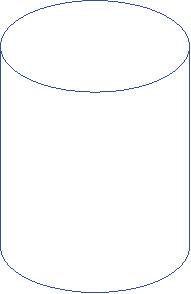Task 3

r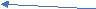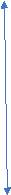h

Let the proposed can be as shown in the figure above, where r is the radius of the base, and h is the height, then we deduce that the volume of the can V is given by:

$V=\pi {r}^{2}h$

Or

$\pi {r}^{2}h=330$

(1)

Also, the total surface area of this can A is given by:

$A=2\pi {r}^{2}+2\mathit{\pi rh}$

(2)

From relation (1) we have $h=\frac{330}{\pi {r}^{2}}$

which when substituted in relation (2) we obtain:

$A=2\pi {r}^{2}+2\mathit{\pi r}×\frac{330}{\pi {r}^{2}}$

Or

$A=2\pi {r}^{2}+\frac{660}{r}$

(3)

Hence to find the minimum area of material needed, we should check whether A given by equation (3), attains a minimum value for some value of r.

Taking the first derivative of this function gives:

${A}^{‘}=4\mathit{\pi r}–\frac{660}{{r}^{2}}$

Then setting A’ equal to zero gives:

So                                                                $r=\sqrt{\frac{165}{\pi }}\approx 3.745\mathit{cm}$

To determine the nature of this local point we apply the second derivative test:

${A}^{‘‘}=4\pi +\frac{1320}{{r}^{3}}$

Which takes positive values for $r=\sqrt{\frac{165}{\pi }}$

since:

${A}^{‘‘}=4\pi +\frac{1320}{\frac{165}{\pi }}=12\pi >0$

Therefore, the minimum area of material needed can be achieved by setting the radius of the can to 3.745cm, and the height h=7.49cm, which gives the minimum surface area of

$A\approx 264.357c{m}^{2}$

To find the minimum and maximum values of p, we use the first derivative as follows:

${p}^{‘}=3{x}^{2}–36x+105$

Then ${p}^{‘}=0$

gives:

$3{x}^{2}–36x+105=0$

$3\left({x}^{2}–12x+35\right)=0$

$\left(x–5\right)\left(x–7\right)=0$

Hence:

Either

Or

To determine the nature of these two points, we apply the second derivative text:

${p}^{‘‘}=6x–36$

So, at the point (5,112) we have:

${p}^{‘‘}=6×5–36=–6<0$

Which implies that the function has a maximum at this point.

Similarly, at the point (7,108) the function has a minimum since:

${p}^{‘‘}=6×7–36=6>0$

A graph of the function is as follows: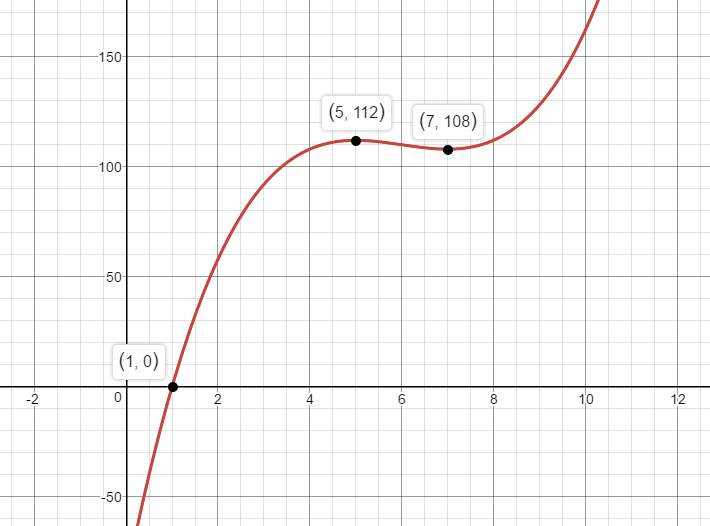Since the horizontal distance AB is the sum of the two segments AD and DB, we can find these two distances by applying Pythagoras Theorem to the triangles ACD and CDB respectively, thus:

From triangle ACD we have:

$A{C}^{2}=A{D}^{2}+C{D}^{2}$

So                                                             $A{D}^{2}={55}^{2}–{20}^{2}=2625$

Which gives                                            $\mathit{AD}\approx 51.24m$

Similarly, applying Pythagoras Theorem on triangle CBD gives:

$C{B}^{2}=C{D}^{2}+B{D}^{2}$

Hence                                                      $B{D}^{2}={30}^{2}–{20}^{2}=500$

Which gives                                            $\mathit{BD}\approx 22.36m$

Therefore,

$\mathit{AB}=\mathit{AD}+\mathit{BD}\approx 51.24+22.36=73.60m$

Conclusion:

The above tasks, and our techniques in approaching them, have shown how powerful a technique calculus can be, by enabling us to understand the behaviour of the functions obtained in these models we applied to solve these tasks.

References:

1. An Introduction To Mathematical Modelling by Michele D Alder 2001.
2. Mathematical Techniques: An Introduction for the Engineering, Physical, and Mathematical Sciences, D W Jordan and P Smith.
3. Desmos.com for graph sketching.

View all

## DMCA / Removal Request

If you are the original writer of this essay and no longer wish to have your work published on UKEssays.com then please:

Related Services

Prices from

S\$203

Approximate costs for:

• 1000 words
• 7 day deliveryHumanity University

Dedicated to your worth and value as a human being!

Related Lectures